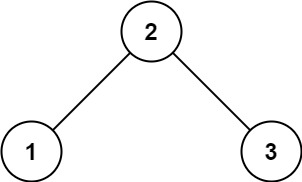# 98. Validate Binary Search Tree | LeetCode Solution

Given the `root` of a binary tree, determine if it is a valid binary search tree (BST).

valid BST is defined as follows:

• The left subtree of a node contains only nodes with keys less than the node's key.
• The right subtree of a node contains only nodes with keys greater than the node's key.
• Both the left and right subtrees must also be binary search trees.

Example 1:```Input: root = [2,1,3]
Output: true
```

Example 2:```Input: root = [5,1,4,null,null,3,6]
Output: false
Explanation: The root node's value is 5 but its right child's value is 4.
```

Constraints:

• The number of nodes in the tree is in the range `[1, 104]`.
• `-231 <= Node.val <= 231 - 1`

/**
* Definition for a binary tree node.
* struct TreeNode {
*     int val;
*     TreeNode *left;
*     TreeNode *right;
*     TreeNode() : val(0), left(nullptr), right(nullptr) {}
*     TreeNode(int x) : val(x), left(nullptr), right(nullptr) {}
*     TreeNode(int x, TreeNode *left, TreeNode *right) : val(x), left(left), right(right) {}
* };
*/
class Solution
{
public:
int rootVal;

bool helper(TreeNode *root, TreeNode *minNode, TreeNode *maxNode)
{

if (!root)
return true;
if (minNode && root->val <= minNode->val || maxNode && root->val >= maxNode->val)
return false;
return helper(root->left, minNode, root) && helper(root->right, root, maxNode);
}

bool isValidBST(TreeNode *root)
{

return helper(root, NULL, NULL);
}
};